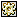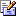Contents Articles Behaviors Books Director News Director Web Sites FAQ Games Mailing Lists News Groups Project Examples Reviews Software Tools Useful Web Sites Utilities Xtras

 Don't miss these#Parse CSV File

 Compatibilities: This item has not yet been rated

Author: MediaMacros (website)

Parses a CSV file or string and returns a list or property list of the results. This is not really useful for very large CSV files as the speed becomes incredibly slow, but works well for shorter ones (IE under about 500 lines or so) This also handles complex CSV like the type exported by Excel.

 --CSV Parser --Copyright 2004 - MediaMacros, Inc. / Chuck Neal --Parses a CSV char by char and returns a linear list or property list of the results --Original C++ version - Copyright Mauricio Piacentini - Tabuleiro --Version History --Created 5/24/2004 --vFile - filename of the CSV OR the text to parse --vCharSep - Character to use as the separator - ",", TAB, etc --vCharQuote - Character to use as the escape quote char - QUOTE, "'", etc --vMaxRecords - Max records to get. 0 or void for all --vNumFields - Number of fields per row.  Script will calculate this itself based on line 1 if set to 0 or void --vFirstLineName - true/false - Denotes wether to use the first line to get names for the property list.  Otherwise each row will be a linear list. on decodeCSV vFile, vCharSep, vCharQuote, vMaxRecords, vNumFields, vFirstLineName   --check the text   if baFileExists(vFIle) then     --import the text         vText = MMI_readTextFile(vFile) & return   else     --assumes this is the text     vText = vFile & return   end if   --are we in "quote mode"   iQuoteMode = 0   --are we in excaped mode   iEscapeMode = 0   --current text   iCurrent = ""   --set the current number of records   iRecords = -1   --check the number of fields     if vNumFields < 1 then vNumFields = 0   --loop through the file   --set the list of records   iFieldNames = []   iRows = []   if vFirstLineName = 1 then     iRow = [:]   else     iRow = []   end if   repeat with x = 1 to vText.char.count     c = vText.char[x]     if c = vCharQuote then       --are we in quote mode?       if iQuoteMode then         if iEscapeMode then           --turn off escape mode           iEscapeMode = false           --add the char           put c after iCurrent         else           --are we escaping, or just finishing the quote?           d = vText.char[x + 1]           if d = vCharQuote then             --turn on escape mode             iEscapeMode = true           else             --turn escape mode off             iQuoteMode = false                       end if         end if       else         iQuoteMode = true       end if     else if c = vCharSep then       --are we in quote mode?       put c after iCurrent       if iQuoteMOde = true then         --not quoting, start new record       else         --is this line 1?         if iRecords < 0 and vFirstLineName = true then           iFieldnames.add(iCurrent)         else           if vFirstLineName <> 1 and iRecords < 0 then             iRecords = 0           end if           --add the content           if iRow.count < vNumFields or vNumFields < 1 then             --only add if we have not exceded the number of allowed fields per row             if vFirstLineName = 1 then               iRow.addProp(iFieldNames[iRow.count + 1], iCurrent)             else               iRow.add(iCurrent)             end if           end if         end if         --reset current         iCurrent = ""       end if     else if c = numToChar(10) then       --new line.  If its in the quote use it, if not, then new record       if iQuoteMode then         --add the newline         put c after iCurrent       else         --not quoting, start new record         --is this line 1?         if iRecords < 0 and vFirstLineName = true then           iFieldnames.add(iCurrent)           if vNumFields = 0 then             vNumFields = iFieldnames.count           end if         else           if vFirstLineName <> 1 and iRecords < 0 then             iRecords = 0           end if           --add the content           if vFirstLineName = 1 then             iRow.addProp(iFieldNames[iRow.count + 1], iCurrent)           else             iRow.add(iCurrent)           end if           --add the row           iRows.add(iRow)           --check the number of fields           if vNumFields = 0 then             vNumFields = iRow.count           end if                      if vFirstLineName = 1 then             iRow = [:]           else             iRow = []           end if           iRecords = iRows.count         end if         --get the field count if needed                  iRecords = iRows.count         --reset current         iCurrent = ""                  if iRecords > vMaxRecords and vMaxRecords > 0 then           return iRows         end if         --turn off escape and quote         iEscapeMode = 0         iQuoteMode = 0                     end if     else if c = numToChar(13) then       if iQuoteMode then         --add the carrier return if in quote mode only         put c after iCurrent       end if     else       put c after iCurrent     end if   end repeat   return iRows end on MMI_readTextFile vFilePath   if string(vFilePath) = "" then     --get a new file     return ""   end if      --verify it exists   if not baFileExists(vFilePath) then     MMI_ReportError(0, "File Does Not Exist")     return ""   end if      fx = new(xtra "fileIO")   if objectp(fx) then     --open in read mode     fx.openFile(vFilePath, 1)     iText = fx.readFile()     fx.closeFile()     fx = void     return iText   end if   return "" end

 Contact MMI 36 South Court Sq Suite 300 Newnan, GA 30263 USASend e-mail